MORE IN Structural Analysis - 2
MU Civil Engineering (Semester 5)
Structural Analysis - 2
December 2015
Total marks: --
Total time: --
INSTRUCTIONS
(1) Assume appropriate data and state your reasons
(2) Marks are given to the right of every question
(3) Draw neat diagrams wherever necessary

1 (a) (i) Carry over factor and Distributed factor.
2 M
1 (a) (ii) Shape factor and load factor.
2 M
1 (a) (iii) Relative stiffness and modified stiffness.
2 M
1 (a) (iv) Flexibility coefficient and stiffness coefficient.
2 M
1 (a) (v) Plastic hinge and mechanism.
2 M
1 (b) Determine shape factor of diamond section of the beam as shown in figure.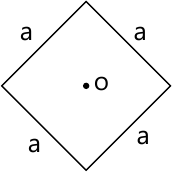4 M
1 (c) Determine the degree of static and kinematic indeterminacy of the following structures. Neglect axial deformation in flexural members and indicate joint displacement.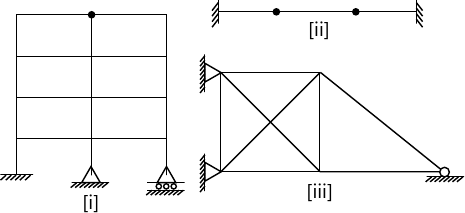6 M

2 (a) The members of a steel bent frame are subjected to temperature variation as shown in fig. Determine the vertical deflection at free end 'C' considering axial deformation in member. Take depth of each member as 500 mm and α=12×10-6/°C.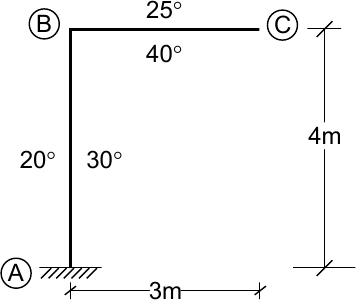6 M
2 (b) Develop the stiffness matrix for prismatic member AB with reference to the co-ordinates as shown in fig.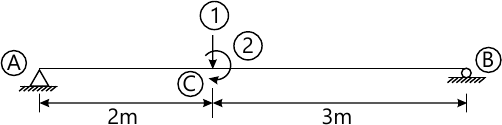6 M
2 (c) Analyse the beam loaded as shown in fig. by Theorem of three moments. Draw BMD.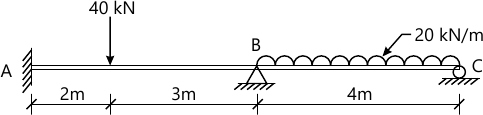8 M

3 (a) Analyse the beam loaded as shown in fig. by Flexibility method and draw BMD.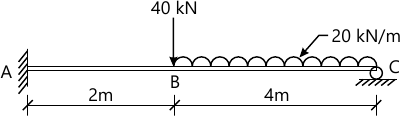10 M
3 (b) Analyse the beam loaded as shown in fig. by Stiffness method and draw BMD.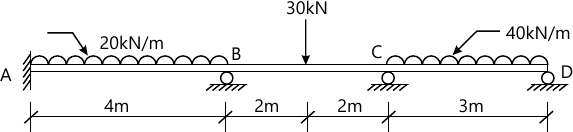10 M

4 (a) Analyse the rigid jointed plane frame as shown in figure, using stiffness method or by MDM. Also draw SFD, BMD and deflected shape.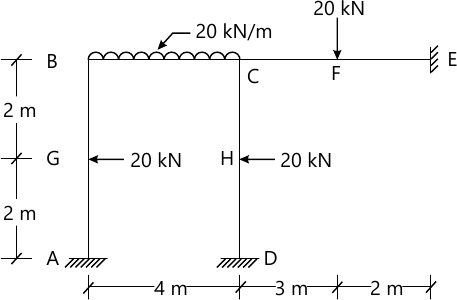12 M
4 (b) Find the reactions at support and draw BMD for a two hinged parabolic arch loaded as shown in figure.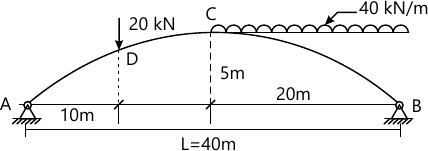8 M

5 (a) Calculate the plastic moment carrying capacity requires for the continuous beam with working load as shown in figure.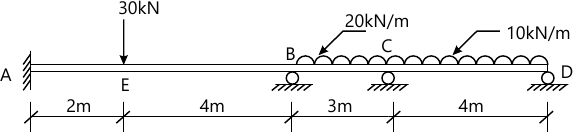8 M
5 (b) Analyse the rigid jointed plane frame as shown in figure, using Flexibility method. Also draw SFD, BMD and deflected shape.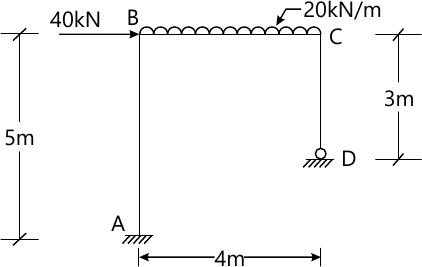12 M

6 (a) Analyse the rigid jointed plane frame as shown in figure, using Slope deflection method, Also draw SFD, BMD and deflected shape.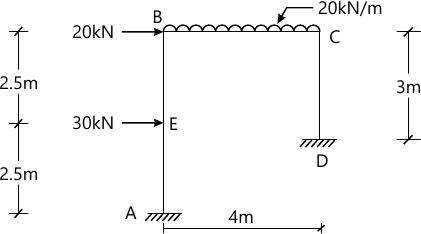12 M
6 (b) Using Force method, analyse the pin jointed pane frame loaded and supported as shown in figure. Take AE=Constant for all members.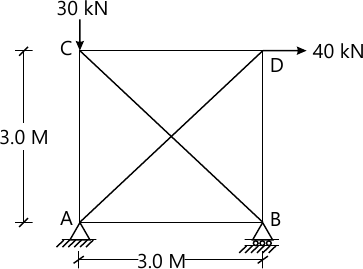8 M

More question papers from Structural Analysis - 2